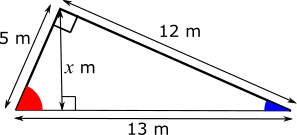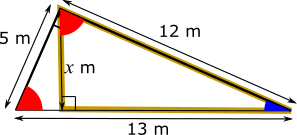#### You may also like### Building Tetrahedra

Can you make a tetrahedron whose faces all have the same perimeter?A 1 metre cube has one face on the ground and one face against a wall. A 4 metre ladder leans against the wall and just touches the cube. How high is the top of the ladder above the ground?Four rods are hinged at their ends to form a convex quadrilateral. Investigate the different shapes that the quadrilateral can take. Be patient this problem may be slow to load.

# Slide Height

##### Age 14 to 16 ShortChallenge Level
Using area
On this diagram, the height we are looking for is labelled $x$ m.There are two ways to view area of the triangle. One is that it has a base of 12 and perpendicular height of 5, and so its area is $\frac{1}{2}\times5\times12 =30$m$^2$.
The other way we can view it is with a base of 13 and perpendicular height of $x$ and so it also has area $\frac{1}{2}\times13x$. We can equate these two areas to get that:
$\frac{13}{2}x = 30 \Rightarrow x=\frac{30\times2}{13}=4.62$m to the nearest cm

Using similar triangles
The height must make a right angle with the ground, which is shown in the diagram below. Also on the diagram, the red angle, the blue angle and a right angle must add up to 180$^{\text{o}}$, because the angles in a triangle add up to 180$^{\text{o}}$, and they are the angles in the triangle made by the slide and the ground.The triangle outlined in gold in the diagram below is also a right-angled triangle. It contains the blue angle and a right angle, so, to add up to 180$^{\text{o}}$, the third angle must be the same as the red angle.This means that the triangle made by the slide and the ground must be similar to the golden triangle.

The hypotenuse of the triangle made by the slide and the ground is 13 m, and the hypotenuse of the golden triangle is 12 m. So the sale factor between the two triangles is $\frac{12}{13}$.

The height, $x$ m, corresponds to the 5 m side of the larger triangle (the ladder). So the height is $\dfrac{12}{13}$ of 5, which is 4.61538..., which is 4.62 m to the nearest cm.
You can find more short problems, arranged by curriculum topic, in our short problems collection.